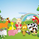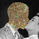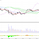TradingView# Linear Quadratic Convergence Divergence Oscillator3033 views
Introduction

I inspired myself from the MACD to present a different oscillator aiming to show more reactive/predictive information. The MACD originally show the relationship between two moving averages by subtracting one of fast period and another one of slow period. In my indicator i will use a similar concept, i will subtract a quadratic least squares moving average with a linear least squares moving average of same period, since the quadratic least squares moving average is faster than the linear one and both methods have low-lag this will result in a reactive oscillator.

LQCD In Details

A quadratic least squares moving average try to fit a quadratic function (parabola) to the price by using the method of least squares, the linear least squares moving average try to fit a line. Non-linear fit tend to minimize the sum of squares in non-linear data, this is why a quadratic method is more reactive. The difference of both filters give us an oscillator, then we apply a simple moving average to this oscillator to provide the signal line, subtracting the oscillator and its signal line give us the histogram, those two last steps are the same used in the MACD .

Length control the period of the quadratic/linear moving average. While the MACD use a signal line for plotting the histogram i also added the option to plot the momentum of the quadratic moving average instead, the result is smoother and reduce irregularities, in order to do so just check the differential option in the parameter box.

The period of the signal line and the momentum are both controlled by the signal parameter.

A predictive approach can be made by subtracting the histogram with the signal line, this process make the histogram way more predictive, in order to do so just check the predictive histogram option in the parameter box.

Predictive histogram with simple histogram option. The differential mode can also be used with the predictive parameter, this result in a smoother but less reactive prediction.

Information Interpretation

The amount of information the MACD can give us is high. We can use the histogram as signal generator, or the if the oscillator is over/under 0, combine the oscillator/signal line with histogram, combinations can provide various systems. Some traders use the histogram as signal generator and use the cross between the histogram and the signal line as a stop signal, this method can avoid some whipsaw trades. The study of divergences with the price is also another method.

Conclusion

This oscillator aim to show the same amount of information as the MACD with a similar calculation method but using different kind of filters as well as eliminating the need to use two separates periods for the moving averages calculation, its still possible to use different periods for the quadratic/linear moving average but the results can be less accurate. This indicator can be used like the MACD .

Open-source Skript

Ganz im Sinne von TradingView hat der Autor dieses Skripts es als Open-Source veröffentlicht, damit Trader es verstehen und überprüfen können. Ein Hoch auf den Autor! Sie können es kostenlos verwenden, aber die Wiederverwendung dieses Codes in einer Publikation unterliegt den Hausregeln. Sie können das Skript den Favoriten hinzufügen, um es auf dem Chart zu verwenden.

Möchten Sie dieses Skript auf einem Chart verwenden?
Patreon: https://www.patreon.com/alexgrover

Become a Patreon and get access to exclusive technical indicators!

You can also check out some of the indicators I made for luxalgo : https://www.tradingview.com/u/LuxAlgo/#published-scripts

## KommentareThat very cool Alex; I love the predictive items; thanks for shared in public =D
AntwortenICEKI
@ICEKI, You are very welcome :) I don't consider sharing in private, i love open source.
AntwortenVery nice Alex, I look forward to messing around with this. Are there any other types of LSMAs that TradingView doesn't provide that could be realised in Pine?
Antwortenchrysopoetics
@chrysopoetics, Thanks for your support, feel free to mess around with it and consider sharing your findings :) If by LSMAs you mean rolling regression of different order then i don't know, the linear and quadratic are the only one i saw in tv, the quadratic regression is a polynomial of order 2 and its still fairly hard to code, a cubic regression (order 3) would be harder, and a quartic (order 4) is certainly not interesting to code.

In theory it would be possible to simplify any regression calculation using the rescaling i method i showed in my previous articles, the only thing to do is to compute the function of the polynomial and rescale it to the price. I tried this by rescaling a parabola to the price but it wasn't working well. I will consider searching a way to compute higher order polynomials.
AntwortenI would like to propose you as a teacher in a math´s corner of TW maybe with videos too,...
Really it is fantastic your work and it can be appelling in order to learn math (not necessary trading)
Antworten